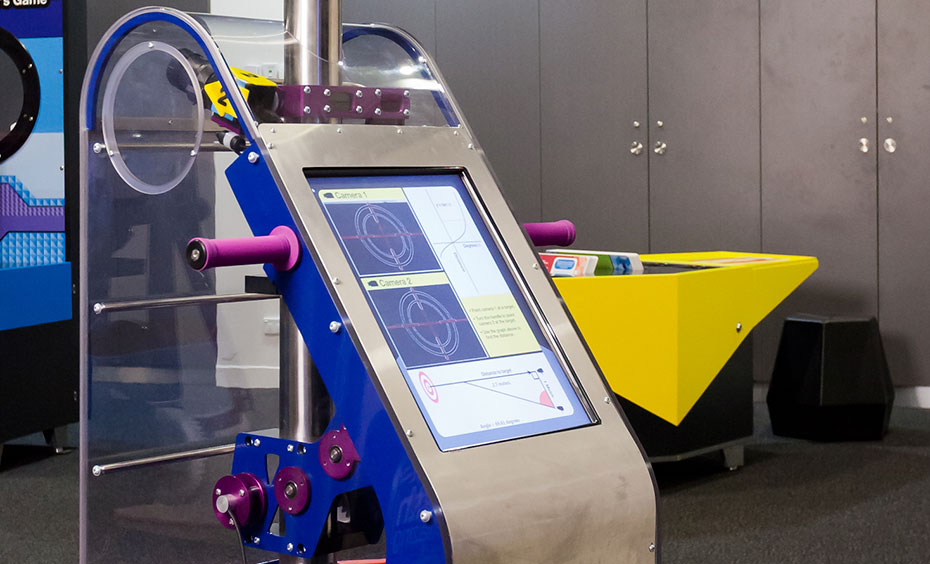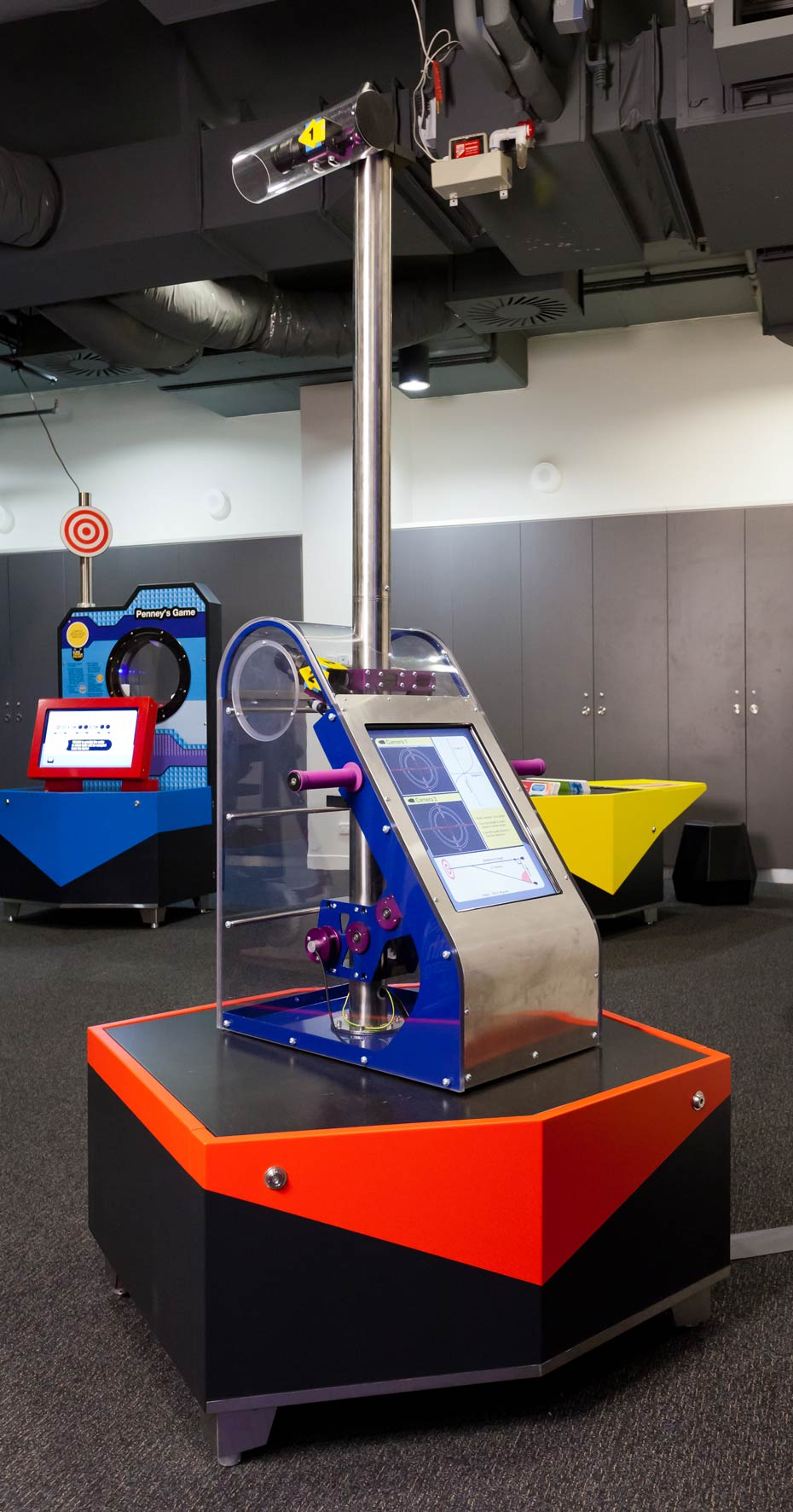# Range FinderThe trigonometry function of 'tan' can be used to find the distance to a target.

## How it works

Move the Camera 2 to focus on a series of targets and check the screen to see the distance of each target and how the distance is being calculated using the function of 'tan'.

## Things to try or ask around the exhibit

• How is distance to the target calculated?
• As you use the exhibit, what do you notice on the graph showing tan θ and the distance to the target?
• Which part of the exhibit's structure represents the right angle in the theoretical triangle?

## Background

While a tape measure is handy for measuring the distance of an object, if that object is very far away, or very tall, you can use the maths of triangulation (especially the trigonometry function tan θ) to determine distances quite quickly.

In the exhibit, the target and Cameras 1 and 2 create an imaginary right angle triangle. As you tilt Camera 2, the computer detects the changing angle (θ). The distance between the two cameras (adjacent side to θ) stays fixed at 1 metre, so the computer can calculate the opposite side of θ, which is also the unknown distance to the target:

tan θ =

opposite side (distance to target)